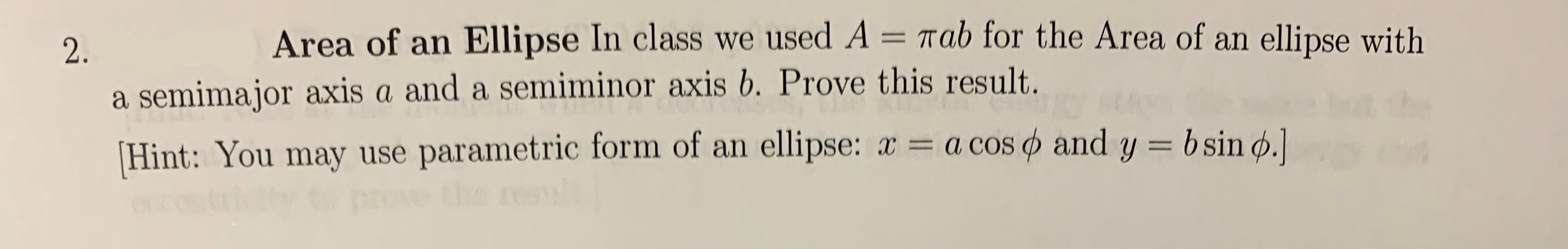# Area of an Ellipse In class we used A = Tab for the Area of an ellipse with2.a semimajor axis a and a semiminor axis b. Prove this result.Hint: You may use parametric form of an ellipse: x a cos and y = bsin d.]

Question
2 viewshelp_outlineImage TranscriptioncloseArea of an Ellipse In class we used A = Tab for the Area of an ellipse with 2. a semimajor axis a and a semiminor axis b. Prove this result. Hint: You may use parametric form of an ellipse: x a cos and y = bsin d.] fullscreen
check_circle

star
star
star
star
star
1 Rating
Step 1

Let the area of an ellipse is denoted by A.

Derive the equation of an ellipse by the given parametric equation.

Step 2

By calculating the area of the quarter of an ellipse and then multiply it by 4 will get us the desired ...

### Want to see the full answer?

See Solution

#### Want to see this answer and more?

Solutions are written by subject experts who are available 24/7. Questions are typically answered within 1 hour.*

See Solution
*Response times may vary by subject and question.
Tagged in

### Other